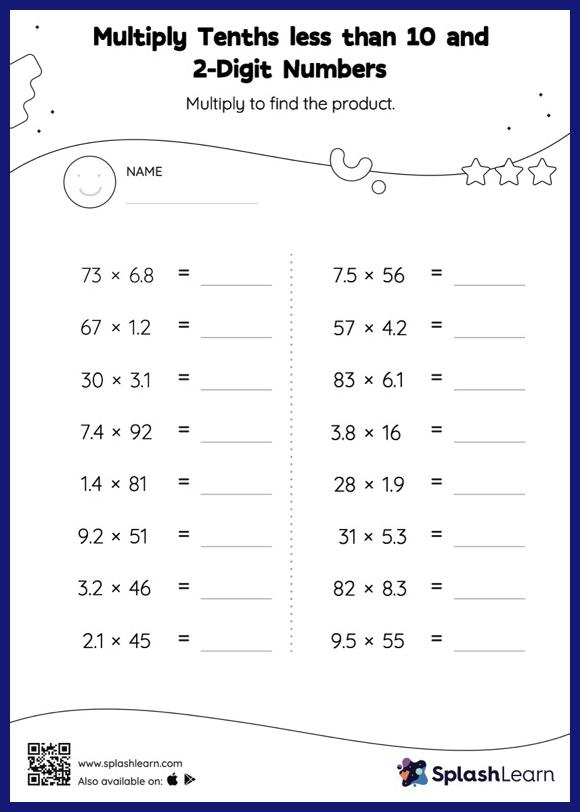# Multiply Tenths less than 10 and 2-Digit Numbers: Horizontal Multiplication Worksheet

Home > Multiply Tenths less than 10 and 2-Digit Numbers: Horizontal MultiplicationWhen multiplying a decimal by a two-digit number, students can omit the decimal point and add it when they write the answer. Learners have numerous opportunities to put this idea into practice with this multiply tenths less than 10 and 2-digit numbers worksheet. As the worksheet uses a horizontal format, it allows for more creativity when it comes to how the student solves the problem. In contrast, in the vertical format, students usually employ the standard method to solve.